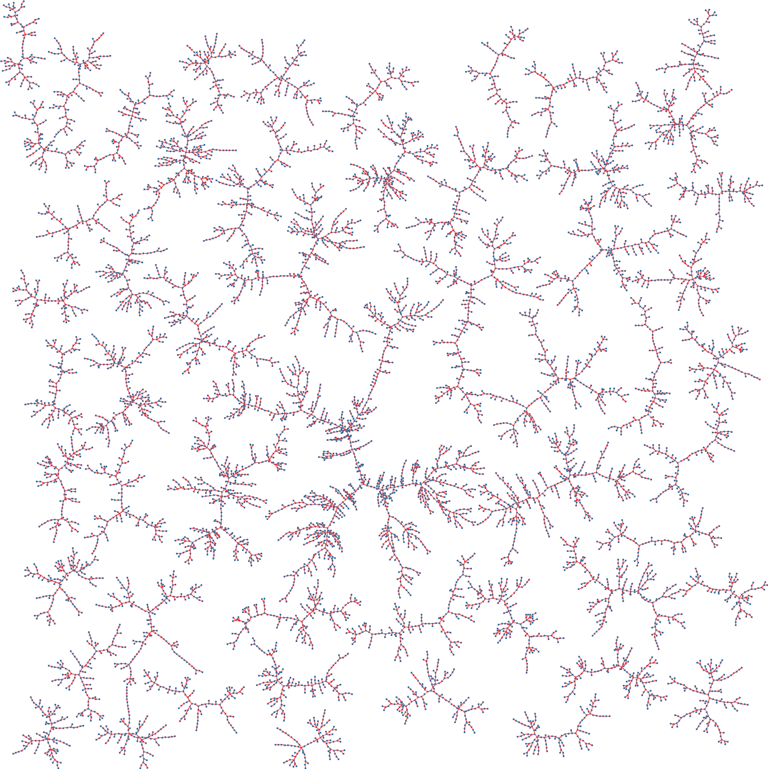## The CHM92 algorithm

Dr. Ilan Schnell, 2008

This article illustrates an algorithm by Z. J. Czech, G. Havas and B.S. Majewski which is described in their 1992 paper "An optimal algorithm for generating minimal perfect hash functions" which appeared in Information Processing Letters, 43(5):257-264, 1992.

A perfect hash function of a certain set S of keys is a hash function which maps all keys in S to different numbers. That means that for the set S, the hash function is collision-free, or perfect. Further, a perfect hash function is called minimal when it maps n keys to n consecutive integers, usually in the range from 0 to n-1.

The algorithm works like this:

1. You have K keys, that you want to perfectly hash against some desired hash values.

2. Choose a number N larger than K. This is the number of vertices in a graph G, and also the size of the resulting table G.

3. Pick two random hash functions f1, f2, that return values from 0..N-1.

4. Now, for all keys, you draw an edge between vertices f1(key) and f2(key) of the graph G, and associate the desired hash value with that edge.

5. Check if G is acyclic; if no, go back to step 2.

6. Assign values to each vertex such that, for each edge, you can add the values for the two vertices and get the desired (hash) value for that edge. This task is easy, because the graph is acyclic. This is done by picking a vertex, and assigning it a value of 0. Then do a depth-first search, assigning values to new vertices so that they sum up properly.

7. f1, f2, and the vertex values of G now make up a perfect hash function.

Suppose our keys are the abbreviations for the 50 states, and we which to map those to the consecutive integers from 0 to 49. We will first explain how the graph works, under the assumption that we have found a perfect hash function and afterwards explain how the algorithm itself works. When the algorithm was completed, we found N = 66. There are of course many ways to the two random hash functions f1 and f2 could have been created. In this example we have used the type of random hash functions described in the original paper, and after the algorithm completed, we found:

``````f1(key) = 15 * key + 29 * key   (mod N)
f2(key) = 50 * key + 61 * key   (mod N)
``````

Where `key` and `key` represent the integer (ASCII) value of the first and second letter in the key. For example:

``````key      f1   f2
----------------
AL  (0)  11   32
CO  (5)  62   51
SD (40)  49   48
TN (41)  24   48
WY (49)  58   11
``````

Note that these hash functions return values in the range 0 to 65 for any keyword with two letters. Note also that even for the set of keys, the functions are not unique, e.g. f2(`SD`) = f2(`TN`) = 48. Having established the two hash functions f1 and f2 (step 3), we now draw the edges between the vertices f1(key) and f2(key). The picture shows all the vertices and edges. For example the is an edge for the state Alabama `AL`, with desired hash value 0, between vertices 11 and 32.

Since the graph is acyclic, we are in a position to assign values for each vertex in such a way that edge value, i.e. the desired hash value, can be calculated by adding the two vertex values. For example for the state Alabama, we get 32 + 34 = 66 = 0 (mod 66) as desired. Or for Colorado `CO` we get 7 + 64 = 71 = 5 (mod 66) as desired. We can therefore just look up the vertex values in a table G, add the values of G and hence obtain the desired hash value.

``````perfect_hash(key) = G[f1(key)] + G[f2(key)]    (mod N)

``````

Now that we understand how the perfect hash values are connected to the vertex values of the graph, one question remains. That is how did we arrive at the vertex values. Here, the key point is that the graph has to be acyclic. Once we found an acyclic graph, we assign (randomly) one vertex of a tree to the value 0, and then traverse the tree (using a depth-first search) such that the vertex values sum up to the edge values correctly. This would not be so easy if the graph was cyclic. Most computing time is spend to find a graph which is acyclic. This is also the reason N needs to be increased after enough failures. The more vertices we have, the easier it will be to randomly draw edges and not create loops. Of course we don't want the N to be too big, because this is the size of the resulting table G. In practice, an a graph with twice as many vertices as keys can be found rather quickly, even if N is quite large. In other words, a random graph with N vertices and E edges is likely to be cyclic if N < 2E and more likely to be acyclic if N > 2E.The picture shows a random graph obtained by the algorithm for 100000 keys. Only trees with more than 100 vertices are shown in the picture and the largest tree in the graph has about 1300 vertices.